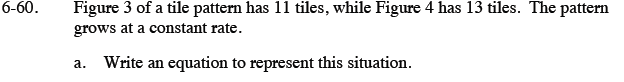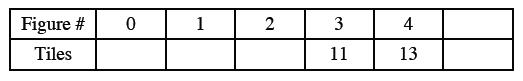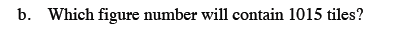### Home > CC3 > Chapter 6 > Lesson 6.2.2 > Problem6-60

6-60.
1. Figure 3 of a tile pattern has 11 tiles, while Figure 4 has 13 tiles. The pattern grows at a constant rate. Homework Help ✎

1. Write an equation to represent this situation.

2. Which figure number will contain 1015 tiles?Make a table for the pattern. Use the table to write your equation.How much does the number of tiles increase as the figure number increases?

y = 2x + 5Let y = 1015 in the equation. Then solve for x.